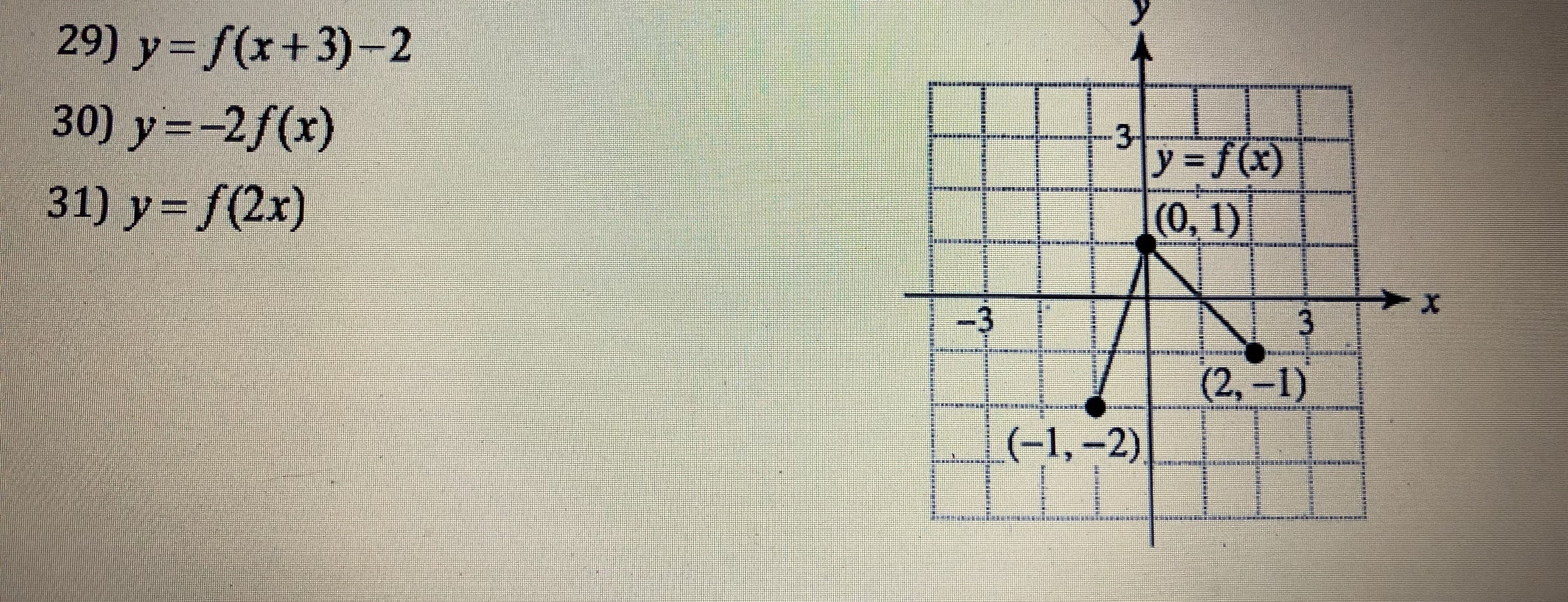29) y=f(x+3)-230) y=-2f(x)31) y-f(2x)

Question

For 31help_outlineImage Transcriptionclose29) y=f(x+3)-2 30) y=-2f(x) 31) y-f(2x) fullscreen
Step 1

From the given diagram the points of the graph of y= f(x) are (–1,–2),(0,1) and (2,–1).

Given function is y= f(2x).

Note that, f(kx) stretches/ shrinks f(x) horizontally. That is, y=f(kx) is the graph of f(x) horizontally shrunk or compressed by dividing each of it’s x - coordinates by k.

Step 2

For transformation of graph of the function y=f(x) to y= f(2x) divide each of x - coordinates by 2.

Then the coordinates of the graph of the function y= f(2x) are (–0.5,–2), (0,1) and (1,–1).

Step 3

Plot these points and connect them with a smooth curve.

The graph of the funct...

Want to see the full answer?

See Solution

Want to see this answer and more?

Our solutions are written by experts, many with advanced degrees, and available 24/7

See Solution
Tagged in

Algebra